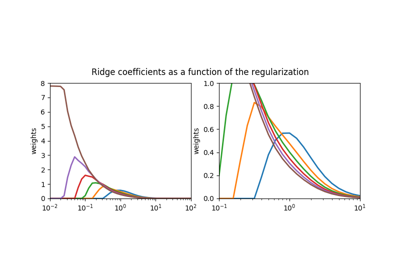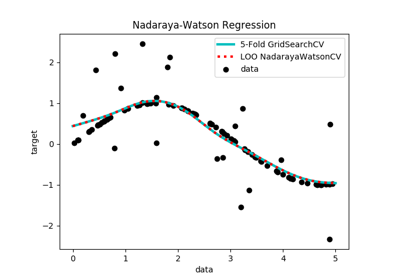# Examples¶

## General Examples¶

General purpose and introductory examples for mcmodels.

## Modified Scikit-Learn Examples¶

The following examples are modified examples from the package Scikit-Learn licensed under the New BSD (3-clause) License reproduced in the each of the following scripts:Plot NonnegativeRidge coefficients as a function of regularizationNonnegativeLinear Least Squares and NonnegativeRidge Regression Variance